field with some ranking property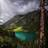Hi everyone,

First message here, new to Ninox and to the board ^^

In a database, I would like to be able to make some kind of “rank system” among the entries.

For example, is there is 50 entries, is there a way to rank them from 1 to 50. It would function like a board, where other entries are automatically pushed “down” when one entry goes up.

I hope my explanation is clear enough.

I have searched for a bit, but I don’t know out to do this, or if it is possible.

Best regards everyone,

Charlie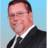Hey Charlie.. Welcome..   Sure..  Simply create a table .. and add a "Rank" column..    Then.. create a view .. and order it by the Rank.. (ascending / descending).. depending on your needs.

Question.. How would the "rank" be set?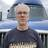It's possible, but the only way I can see it working is with two rank fields so you can compare. This scenario is crying for a dialog box that allows input.

You can attach this to a button:

if Rank1 != Rank2 then
let numOldRank := Rank1;
let numNewRank := Rank2;
if numOldRank < numNewRank then
for c in (select Table1)[Rank1 > numOldRank and Rank1 <= numNewRank] do
c.(Rank1 := c.Rank1 - 1);
c.(Rank2 := c.Rank2 - 1);
Rank1 := numNewRank;
openTable("Table1")
end
else
if numOldRank > numNewRank then
for c in (select Table1)[Rank1 < numOldRank and Rank1 >= numNewRank] do
c.(Rank1 := c.Rank1 + 1);
c.(Rank2 := c.Rank2 + 1);
Rank1 := numNewRank;
openTable("Table1")
end
end
end
endI just wanted to update my post with a little more explanation. I don't know if this is what is referred to as a "Use Case", but the task is to auto update Rankings in a table. If you change a record's rank, you will by definition displace another record. This means if you do it manually you will have to update the ranks of both records and that is if they are consecutive. If they aren't you'll have to update more ranks. Add to that, updating the ranks does not update the view. To do that you would need to also click the refresh icon.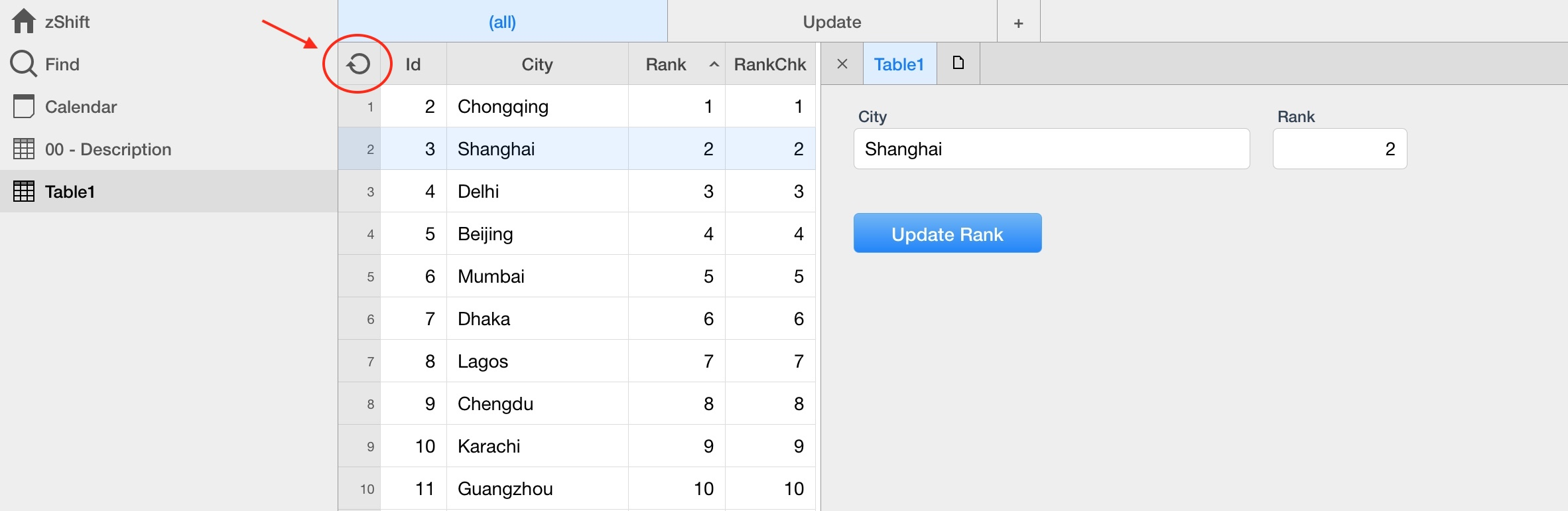The code I posted previously was a mashup of an idea I had and my punt solution. I've changed it so "Rank" is the field you update and "RankChk", while necessary, can be hidden. I've attached an image of the code because it's easier to read with the indentation, at least it is to me.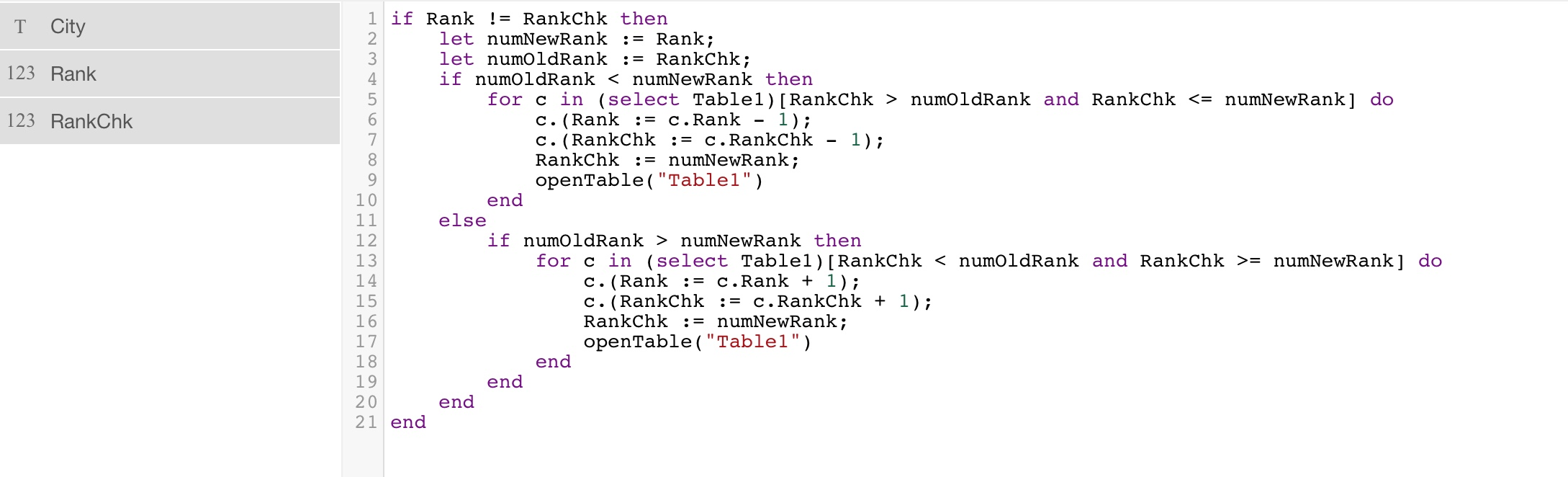This is the formula for the button. All you have to do is change the rank number in the form and click "Update Rank" and the work is done. Here's the code for copy/paste.

if Rank != RankChk then
let numNewRank := Rank;
let numOldRank := RankChk;
if numOldRank < numNewRank then
for c in (select Table1)[RankChk > numOldRank and RankChk <= numNewRank] do
c.(Rank := c.Rank - 1);
c.(RankChk := c.RankChk - 1);
RankChk := numNewRank;
openTable("Table1")
end
else
if numOldRank > numNewRank then
for c in (select Table1)[RankChk < numOldRank and RankChk >= numNewRank] do
c.(Rank := c.Rank + 1);
c.(RankChk := c.RankChk + 1);
RankChk := numNewRank;
openTable("Table1")
end
end
end
endHi Mconneen and slowwagon,

Mconneen, what I was after was something very close to the answer of slowwagon, granted my formulation/question might have been better. It was all about “automagically” “displacing” entries below if an entry went up manually.

slowwagon, Whoaaah! That is a very complete and thorough answer, thank you very much, because I may generally understand your code, but I would have been totally incapable of doing this all by myself ^^'

And it works! Thank you thank you!

Best regards to you both,

Charlie